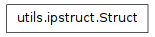utils.io

utils.jsonutil

# utils.ipstruct¶

## Module: utils.ipstruct¶

Inheritance diagram for IPython.utils.ipstruct:A dict subclass that supports attribute style access.

Authors:

• Fernando Perez (original)
• Brian Granger (refactoring to a dict subclass)

## Struct¶

class IPython.utils.ipstruct.Struct(*args, **kw)

Bases: dict

A dict subclass with attribute style access.

This dict subclass has a a few extra features:

• Attribute style access.
• Protection of class members (like keys, items) when using attribute style access.
• The ability to restrict assignment to only existing keys.
• Intelligent merging.
__init__(*args, **kw)

Initialize with a dictionary, another Struct, or data.

Parameters : args : dict, Struct Initialize with one dict or Struct kw : dict Initialize with key, value pairs.

Examples

```>>> s = Struct(a=10,b=30)
>>> s.a
10
>>> s.b
30
>>> s2 = Struct(s,c=30)
>>> s2.keys()
['a', 'c', 'b']
```
allow_new_attr(allow=True)

Set whether new attributes can be created in this Struct.

This can be used to catch typos by verifying that the attribute user tries to change already exists in this Struct.

clear

D.clear() -> None. Remove all items from D.

copy()

Return a copy as a Struct.

Examples

```>>> s = Struct(a=10,b=30)
>>> s2 = s.copy()
>>> s2
{'a': 10, 'b': 30}
>>> type(s2).__name__
'Struct'
```
dict()
static fromkeys()

dict.fromkeys(S[,v]) -> New dict with keys from S and values equal to v. v defaults to None.

get

D.get(k[,d]) -> D[k] if k in D, else d. d defaults to None.

has_key

D.has_key(k) -> True if D has a key k, else False

hasattr(key)

hasattr function available as a method.

Implemented like has_key.

Examples

```>>> s = Struct(a=10)
>>> s.hasattr('a')
True
>>> s.hasattr('b')
False
>>> s.hasattr('get')
False
```
items

D.items() -> list of D’s (key, value) pairs, as 2-tuples

iteritems

D.iteritems() -> an iterator over the (key, value) items of D

iterkeys

D.iterkeys() -> an iterator over the keys of D

itervalues

D.itervalues() -> an iterator over the values of D

keys

D.keys() -> list of D’s keys

merge(__loc_data__=None, _Struct__conflict_solve=None, **kw)

Merge two Structs with customizable conflict resolution.

This is similar to update(), but much more flexible. First, a dict is made from data+key=value pairs. When merging this dict with the Struct S, the optional dictionary ‘conflict’ is used to decide what to do.

If conflict is not given, the default behavior is to preserve any keys with their current value (the opposite of the update() method’s behavior).

Parameters : __loc_data : dict, Struct The data to merge into self __conflict_solve : dict The conflict policy dict. The keys are binary functions used to resolve the conflict and the values are lists of strings naming the keys the conflict resolution function applies to. Instead of a list of strings a space separated string can be used, like ‘a b c’. kw : dict Additional key, value pairs to merge in

Notes

The __conflict_solve dict is a dictionary of binary functions which will be used to solve key conflicts. Here is an example:

```__conflict_solve = dict(
func1=['a','b','c'],
func2=['d','e']
)
```

In this case, the function func1() will be used to resolve keys ‘a’, ‘b’ and ‘c’ and the function func2() will be used for keys ‘d’ and ‘e’. This could also be written as:

```__conflict_solve = dict(func1='a b c',func2='d e')
```

These functions will be called for each key they apply to with the form:

```func1(self['a'], other['a'])
```

The return value is used as the final merged value.

As a convenience, merge() provides five (the most commonly needed) pre-defined policies: preserve, update, add, add_flip and add_s. The easiest explanation is their implementation:

```preserve = lambda old,new: old
update   = lambda old,new: new
add      = lambda old,new: old + new
add_flip = lambda old,new: new + old  # note change of order!
add_s    = lambda old,new: old + ' ' + new  # only for str!
```

You can use those four words (as strings) as keys instead of defining them as functions, and the merge method will substitute the appropriate functions for you.

For more complicated conflict resolution policies, you still need to construct your own functions.

Examples

This show the default policy:

```>>> s = Struct(a=10,b=30)
>>> s2 = Struct(a=20,c=40)
>>> s.merge(s2)
>>> s
{'a': 10, 'c': 40, 'b': 30}
```

Now, show how to specify a conflict dict:

```>>> s = Struct(a=10,b=30)
>>> s2 = Struct(a=20,b=40)
>>> conflict = {'update':'a','add':'b'}
>>> s.merge(s2,conflict)
>>> s
{'a': 20, 'b': 70}
```
pop

D.pop(k[,d]) -> v, remove specified key and return the corresponding value. If key is not found, d is returned if given, otherwise KeyError is raised

popitem

D.popitem() -> (k, v), remove and return some (key, value) pair as a 2-tuple; but raise KeyError if D is empty.

setdefault

D.setdefault(k[,d]) -> D.get(k,d), also set D[k]=d if k not in D

update

D.update(E, **F) -> None. Update D from dict/iterable E and F. If E has a .keys() method, does: for k in E: D[k] = E[k] If E lacks .keys() method, does: for (k, v) in E: D[k] = v In either case, this is followed by: for k in F: D[k] = F[k]

values

D.values() -> list of D’s values

viewitems

D.viewitems() -> a set-like object providing a view on D’s items

viewkeys

D.viewkeys() -> a set-like object providing a view on D’s keys

viewvalues

D.viewvalues() -> an object providing a view on D’s values